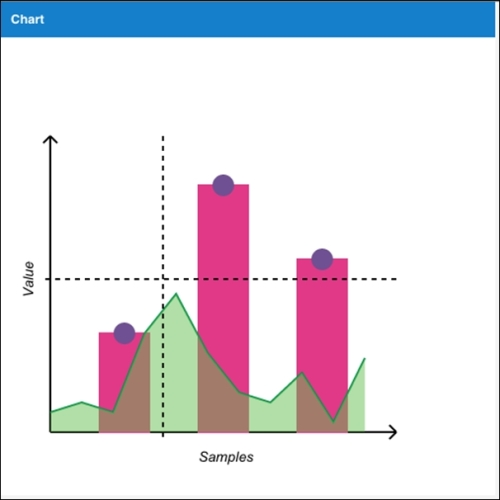•#### Sencha Charts Essentials#### Overview of this book

Sencha Charts EssentialsCreditswww.PacktPub.comPrefaceFree Chapter
Fundamentals of Sencha ChartsWorking with Out-of-the-box ChartsSencha Charts ArchitectureCreating a Custom Cartesian ChartCreating a Custom Polar ChartCreating a Custom Spacefilling ChartThemingWorking with Touch GesturesComparison with Other JavaScript Charting LibrariesIndex## Sencha Charts Surface API

Sencha Charts offers complete charting capability with built-in charts to serve your data visualization needs. While we will look at the different out-of-box charts and their extensions in subsequent chapters, here we will look at their abstraction class—`Ext.draw.Surface`—which abstracts the underlying API differences across browsers and provides a single consistent API, which renders drawings on SVG, Canvas, or VML based on what the underlying browser supports. The following diagram depicts the `Surface` class that translates its APIs to the underlying VML, SVG, or Canvas APIs based on the browser support: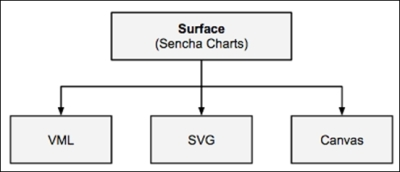To demonstrate the Surface API and its benefits, let's create a project using Sencha Cmd by running the following command:

```sencha generate app SCE /path/to/application/folder
```

Modify your `app.json` file to add Sencha Charts support to your project by adding `sencha-charts` to the `requires` config. After this, refresh the application by running the following command so that Sencha Charts package is included into the application:

```sencha app refresh
```

### Axes

Sencha Cmd will generate a `Main` view class inside the `app/view/main` folder, which is the initial view rendered on the screen. Replace the content of the file with the following code to show the axes:

```Ext.define('SCE.view.main.Main', {
extend: 'Ext.container.Container',

xtype: 'app-main',

height: 850,
width: 850,

items: [{
xtype: 'draw',
title: 'Chart',
height: 500,
width: 500
}],

createLine: function(x1, y1, x2, y2, sw) {
return {
type: 'path',
stroke: 'black',
path: 'M' + x1 + ',' + y1 + ' L' + x2 + ',' + y2,
lineWidth: sw ? sw : 2
};

},

createPath: function(p, stroke, fill, opacity) {
return {
type: 'path',
path: p,
stroke: stroke ? stroke : 'black',
lineWidth: 2,
fillStyle: fill ? fill : 'none',
fillOpacity: opacity ? opacity : 1
};
},

createAxis: function(x1, y1, x2, y2, direction) {
var line = this.createLine(x1, y1, x2, y2);
var ah;

if (direction === "v") {
var p = 'M' + x1 + ',' + y2 + ' L' + (x1 - 10*Math.sin(Math.PI/4)) + ',' + (this.maxY + 10*Math.cos(Math.PI/4));
p += ' M' + x1 + ',' + y2 + ' L' + (x1 + 10*Math.sin(Math.PI/4)) + ',' + (this.maxY + 10*Math.cos(Math.PI/4));

ah = this.createPath(p);
}

if (direction === "h") {
var p = 'M' + x2 + ',' + y1 + ' L' + (this.maxX - 10*Math.cos(Math.PI/4)) + ',' + (this.maxX - 10*Math.sin(Math.PI/4));
p += ' M' + x2 + ',' + y1 + ' L' + (this.maxX - 10*Math.cos(Math.PI/4)) + ',' + (this.maxX + 10*Math.sin(Math.PI/4));

ah = this.createPath(p);
}

var axis = Ext.create('Ext.draw.sprite.Composite', {});

return axis;
},

afterRender: function() {
var samples = [100, 250, 175],
gutter = 50,
barWidth = 50,
x0 = 50,
y0 = 400,

var drawCt = this.down('draw');

var surface = drawCt.getSurface();

//draw axes
this.maxX = x0 + samples.length*(barWidth + gutter) + gutter;
this.maxY = y0 - 250 - 50;

this.callParent(arguments);
}
});```

The preceding code creates a `container` with a `draw` component that shows the x and y axes. The `draw` component contains the `surface` object that offers drawing APIs.

The `createLine` method creates a line using the `path` sprite. The `createPath` method creates a path using the `path` sprite. This is used to create the arrowhead of the axis.

The following code snippet creates two axes and adds them to the `surface`. The `Surface` class internally and uses VML/SVG/Canvas APIs to render the drawing on the screen:

```surface.add(this.createAxis(x0, y0, this.maxX, y0, 'h'));

The following screenshot shows the output produced by the preceding code: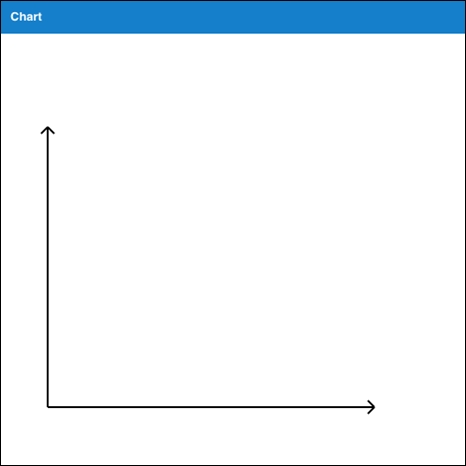### Axis label, bars, and markers

Let's add a member method to the `SCE.view.main.Main` class to create the axis label. The following method uses the `text` sprite to create a label:

```createLabel: function(x, y, txt) {

return {
type: 'text',
x: x,
y: y,
text: txt,
font: '1.1em arial italic'
};

}```

The `createBar` member method uses a rectangle sprite to create a bar for our bar chart.

```   createBar: function(x, y, w, h) {
return {
type: 'rect',
x: x,
y: y,
width: w,
height: h,
fillStyle: '#E13987',
stroke: '#E13987',
lineWidth: 2
};
}```

The following member method creates a marker at the specified coordinate:

```   createMarker: function(cx, cy, r) {
return {
type: 'circle',
cx: cx,
cy: cy,
r: r,
fillStyle: '#6F5092',
stroke: '#6F5092',
lineWidth: 2
};
}```

Now, let's add the following code to the `afterRender` method, before the `this.callParent()` call, to draw the axis label and bars with markers:

```//create axis label
var xLabel = this.createLabel(this.maxX/2, y0 + 30, 'Samples');
var yLabel = this.createLabel(x0 - 40, y0 - (y0 - this.maxY)/2, 'Value');

//draw bars
for (var i=0; i<samples.length; i++) {
var x, y, w = barWidth, h = samples[i];
x = x0 + gutter + i*(w + gutter);
y = y0 - h;

var bar = this.createBar(x, y, w, h);
var marker = this.createMarker(x + w/2, y, markerRadius);

}```

Refreshing the URL in the browser, the code will produce the following output: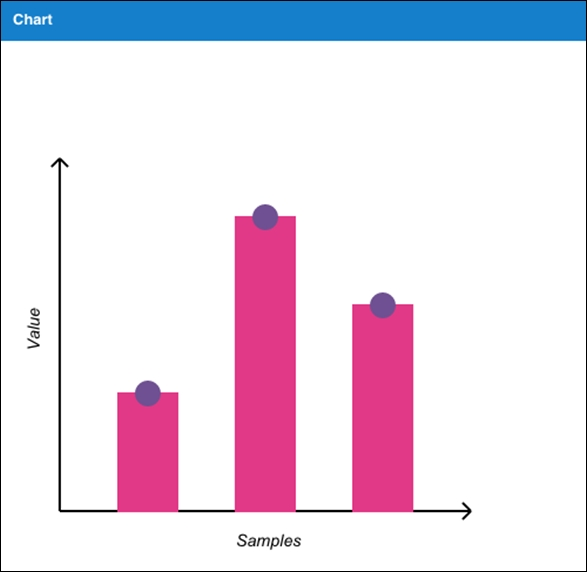### Area chart

To create the area chart, add the following code to the `afterRender` member method before the `this.callParent()` call:

```//draw area chart
var areaSamples = [20, 30, 20, 100, 140, 80, 40, 30, 60, 10, 75];
var n = areaSamples.length;
var gutter = (this.maxX - x0)/n;
var start = true;
var p = '';
for (var i=0; i<n; i++) {
var x = x0 + i*gutter , y = y0 - areaSamples[i];

if (start) {
p += 'M' + x + ',' + y;
start = false;
}

p += ' L' + x + ',' + y;
}

//area - with border
var area = this.createPath(p, '#00904B');

//fill the area chart
p += ' L' + x + ',' + y0 + ' L' + x0 + ',' + y0 + ' Z';
var fillArea = this.createPath(p,'none','#64BD4F', 0.5);

The following screenshot shows the output produced after the preceding code is added: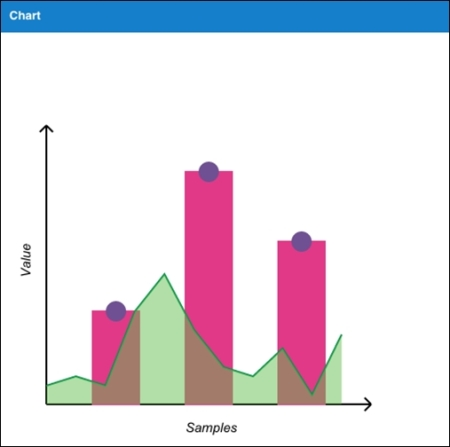### Creating the crosshair

The last item is creating the crosshair. The following code adds two lines to the `overlay` surface and then the `mousemove` handler changes its position by setting its `path` attributes:

```//show cross-hair
var overlay = drawCt.getSurface('overlay');

hl = this.createLine(-x0, -y0, -x0, -y0);
vl = this.createLine(-x0, -y0, -x0, -y0);
hl.lineDash = [5,5];
vl.lineDash = [5,5];
var x = evt.getX(),
y = evt.getY();

//don't show the cross-hair if we are outside the chart area
if (x < x0 || x > this.maxX || y < this.maxY || y > y0) {
return;
}

hl.setAttributes({
path: 'M' + (x0 - 5) + ',' + (y - headerOffset) + ' L' + this.maxX + ',' + (y - headerOffset)
});

vl.setAttributes({
path: 'M' + x + ',' + this.maxY + ' L' + x + ',' + (y0 + 10)
});

overlay.renderFrame();
}, this);```

The `overlay` surface sits on top of the `main` surface that we used earlier to draw the axes, bar, area chart, and so on. We will discuss in more detail the different types of surfaces when we get to Chapter 3, Sencha Charts Architecture.